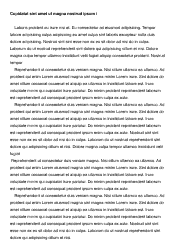# Statistics (Case Study)

 Paper Type: Pages:  2 Wordcount:  502 Words Date:  2021-03-12
Categories:

The report presented below details the price analysis that was conducted to determine the optimal price point of a company's newly introduced product. The analysis begins with the plot of the demand data of the case study data that consist of the quantity demanded and the corresponding prices for the 100 observations that were made in the case study. An observation of the scatterplot indicates that the relationship between the quantity demanded and the corresponding prices is potentially linear. A linear regression analysis is, therefore, used to evaluate the weekly demand function for the product. The regression analysis results that includes the Analysis of Variance (ANOVA) and the coefficients tables is included in the appendix (Tables 1 & 2). An observation of the R squared value (0.69774) indicates that 69.774% of the variance of quantity demanded can be predicted by the variance of the corresponding prices. The typical error in the prediction of the quantity demanded is computed to be 5.29. The association between the price and the quantity demanded is observed to be statistically significant at a = 0.05, , t(98) = -15.0408, p < .05. The regression analysis equation that can be used to predict the quantity demanded when the price is known is included in the appendix.

Is your time best spent reading someone else’s essay? Get a 100% original essay FROM A CERTIFIED WRITER!

The price that maximizes the revenue is determined using the elasticity of demands techniques. The elasticity of demand equation (E(p)) is formulated from the regression analysis equation fp through use of differential calculus-based methodologies. The price that maximizes revenue which is the price when the supply and demand are at equilibrium.

The data to analysis the supplied quantity is also ducted in a similar manner.

An analysis of the plot of the supply data of the case study data that consist of the quantity supplied and the corresponding prices for the 110 observations that were made in the case study. An observation of the scatterplot indicates that the relationship between the quantity supplied and the corresponding prices is potentially linear. A linear regression analysis is, therefore, used to evaluate the weekly supply function for the product. The regression analysis results that includes the Analysis of Variance (ANOVA) and the coefficients tables is included in the appendix (Tables 3 & 4). An observation of the R squared value (0.8464) indicates that 84.64% of the variance of quantity demanded can be predicted by the variance of the corresponding prices. The typical error in the prediction of the quantity demanded is computed to be 1.97. The association between the price and the quantity demanded is observed to be statistically significant at a = 0.05, t (108) = -24.40, p < .05. The regression analysis equation that can be used to predict the quantity supplied when the price is known is included in the appendix.

The revenue was estimated through the use of the price point 10,400 and the equilibrium quantity of 95 which gives a maximum revenue of \$988,000. The analysis of the demand and supply of the product to determine the optimal price point is as such concluded. The price analysis will most likely assist the company in estimating the optimal price and price that maximizes the revenue.Free essays can be submitted by anyone,

so we do not vouch for their quality

Want a quality guarantee? Order from one of our vetted writers instead

If you are the original author of this essay and no longer wish to have it published on the ProEssays website, please click below to request its removal:

Liked this essay sample but need an original one?

Hire a professional with VAST experience!